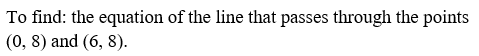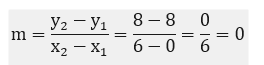# Write an equation of the line that passes through (0, 8)(0, 8) and (6, 8)(6, 8).

Question
1 views

Write an equation of the line that passes through (0, 8)(0, 8) and (6, 8)(6, 8).

check_circle

Given two points (0, 8) and (6, 8)Now, we find the slope of the line as below:...

### Want to see the full answer?

See Solution

#### Want to see this answer and more?

Solutions are written by subject experts who are available 24/7. Questions are typically answered within 1 hour.*

See Solution
*Response times may vary by subject and question.
Tagged in